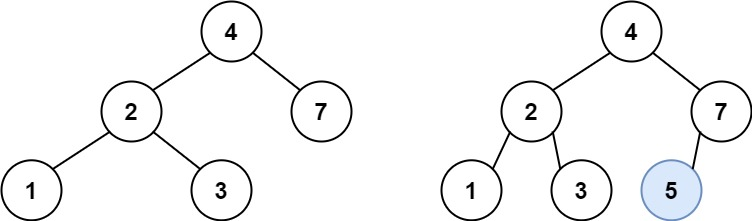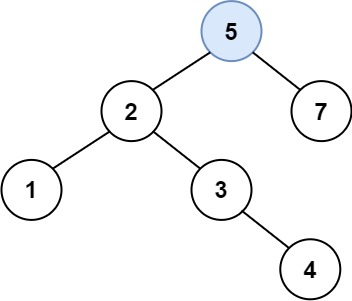Amazon

Microsoft

2020-10-06

## 701. Insert into a Binary Search Tree

### Question:

You are given the `root` node of a binary search tree (BST) and a `value` to insert into the tree. Return the root node of the BST after the insertion. It is guaranteed that the new value does not exist in the original BST.

Notice that there may exist multiple valid ways for the insertion, as long as the tree remains a BST after insertion. You can return any of them.

#### Example 1:``````Input: root = [4,2,7,1,3], val = 5
Output: [4,2,7,1,3,5]
Explanation: Another accepted tree is:
``````#### Example 2:

``````Input: root = [40,20,60,10,30,50,70], val = 25
Output: [40,20,60,10,30,50,70,null,null,25]
``````

#### Example 3:

``````Input: root = [4,2,7,1,3,null,null,null,null,null,null], val = 5
Output: [4,2,7,1,3,5]
``````

Constraints:

• The number of nodes in the tree will be in the range `[0, 104]`.
• `-108 <= Node.val <= 108`
• All the values `Node.val` are unique.
• `-108 <= val <= 108`
• It’s guaranteed that `val` does not exist in the original BST.

### Solution:

There are two ways to perform the binary search: iterative or recursion.
Time Complexity: O(n) where n is the height of the tree

Iterative

``````/**
* Definition for a binary tree node.
* public class TreeNode {
*     int val;
*     TreeNode left;
*     TreeNode right;
*     TreeNode() {}
*     TreeNode(int val) { this.val = val; }
*     TreeNode(int val, TreeNode left, TreeNode right) {
*         this.val = val;
*         this.left = left;
*         this.right = right;
*     }
* }
*/
class Solution {
public TreeNode insertIntoBST(TreeNode root, int val) {
if (root == null) return new TreeNode(val);
TreeNode curr = root;
while(curr != null) {
if (curr.val > val) {
if (curr.left == null) {
curr.left = new TreeNode(val);
break;
}
curr = curr.left;
}
else {
if (curr.right == null) {
curr.right = new TreeNode(val);
break;
}
curr = curr.right;
}
}
return root;
}
}
``````

Recursion

``````/**
* Definition for a binary tree node.
* public class TreeNode {
*     int val;
*     TreeNode left;
*     TreeNode right;
*     TreeNode() {}
*     TreeNode(int val) { this.val = val; }
*     TreeNode(int val, TreeNode left, TreeNode right) {
*         this.val = val;
*         this.left = left;
*         this.right = right;
*     }
* }
*/
class Solution {
public TreeNode insertIntoBST(TreeNode root, int val) {
if (root == null) return new TreeNode(val);
if (root.val > val) {
root.left = insertIntoBST(root.left, val);
}
if (root.val < val) {
root.right = insertIntoBST(root.right, val);
}
return root;
}
}
``````